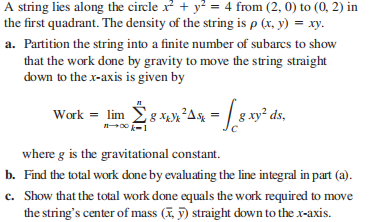### Still have math questions?

Trigonometry
QuestionA string lies along the circle $$x ^ { 2 } + y ^ { 2 } = 4$$ from $$( 2,0 )$$ to $$( 0,2 )$$ in the first quadrant. The density of the string is $$\rho ( x , y ) = x y$$ .

a. Partition the string into a finite number of subarcs to show that the work done by gravity to move the string straight down to the $$x$$ -axis is given by Work $$= \lim _ { n \rightarrow \infty } \sum _ { k = 1 } ^ { n } g x _ { k } y _ { k } ^ { 2 } \Delta s _ { k } = \int _ { C } g x y ^ { 2 } d s$$ , where $$g$$ is the gravitational constant.

b. Find the total work done by evaluating the line integral in part (a).

c. Show that the total work done equals the work required to move the string's center of mass $$( \overline { x } , \overline { y } )$$ straight down to the $$x$$ -axis.

$$\text { (b) } \frac { 16 } { 3 } g \quad \text { (c) Work } = ( \int _ { C } g x y d s ) \overline { y } = g \int _ { C } x y ^ { 2 } d s = \frac { 16 } { 3 } g$$